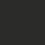# Introduction to Trigonometry $1$

$\textbf{Some History}$

In the $1800$s, when India was still a British colony, a British surveyor stationed not far away from the foothills of the Himalayas sighted an extremely high peak in the distance. When a mathematician calculated its height based on measurements taken from a survey, he realised that it was the world's highest mountain. It was then named Mount Everest, in honour of Sir George Everest, the Surveyor-General of India.

How was the mountain's height measured?

$\bullet$ Firstly, a point $P$ on the ground away from the mountain was chosen.

$\bullet$ Next, the distance $d$ from $P$ to the base of the mountain was measured as precisely as possible (this was extremely difficult to do due to hilly terrain).

$\bullet$ The angle of elevation $\theta$ of the summit from the point $P$ was then measured.

$\bullet$ Using trigonometry, the mountain's height was finally determined.

What, then, is trigonometry? Basically, it is the study of the relationship between angles and lengths.

$\textbf{1. The Trigonometric Ratios}$

a. Defining the trigonometric ratios at acute angles

Let $\theta$ be an acute angle, i.e. $0^{\circ} < \theta < 90^{\circ}$. Draw a right-angled triangle $\triangle ABC$ with $\angle A=\theta$ and $\angle B=90^{\circ}$.

The sine, cosine and tangent ratios at the angle $\theta$ are then defined as follows:

$\sin \theta = \frac{\text{Opposite side}}{\text{Hypothenuse}}=\frac{BC}{AC};$

$\cos \theta = \frac{\text{Adjacent side}}{\text{Hypotenuse}}=\frac{AB}{AC};$

$\tan \theta = \frac{\text{Opposite side}}{\text{Adjacent side}}=\frac{BC}{AB}.$

To remember these definitions more easily, memorise the mnemonic 'toa-cah-soh' or 'soh-cah-toa'.

b. Exercises

$1$. Use the concept of similar triangles to show that the definitions of the three basic trigonometric ratios at an acute angle do not depend on the size of the right-angled triangle drawn.

$2$. Show that, for acute $\theta$, $\cos(90^{\circ}-\theta)=\sin \theta$.

$3$. Construct $\triangle ABC$ such that $\angle B=90^{\circ}, AB=BC=1, AC=\sqrt{2}$. Construct a second $\triangle DEF$ such that $\angle E=90^{\circ}, DE=\sqrt{3},EF=1$ and $DF=2$. Use the triangles to determine the following values:

$\sin 30^{\circ}, \sin 45^{\circ}, \sin 60^{\circ},$

$\cos 30^{\circ}, \cos 45^{\circ}, \cos 60^{\circ},$

$\tan 30^{\circ}, \tan 45^{\circ}, \tan 60^{\circ}.$

$4$. Construct $\triangle ABC$ such that $\angle B=90^{\circ}$ and $\angle A=\theta$. If $\tan \theta = \frac{4}{3}$, find the values of $\sin \theta$ and $\cos \theta$.

$5$. Show that, for acute angle $\theta$, $\sin^{2}\theta +\cos^{2}\theta = 1$.

$\textbf{Details and Assumptions:}$

$\sin^{2}\theta = (\sin\theta)^{2}, \cos^{2}\theta=(\cos\theta)^{2}$.

In the next note, Introduction to Trigonometry $2$ (coming soon!), we will define the three basic trigonometric ratios at other angles.Note by Victor Loh
6 years, 9 months ago

This discussion board is a place to discuss our Daily Challenges and the math and science related to those challenges. Explanations are more than just a solution — they should explain the steps and thinking strategies that you used to obtain the solution. Comments should further the discussion of math and science.

When posting on Brilliant:

• Use the emojis to react to an explanation, whether you're congratulating a job well done , or just really confused .
• Ask specific questions about the challenge or the steps in somebody's explanation. Well-posed questions can add a lot to the discussion, but posting "I don't understand!" doesn't help anyone.
• Try to contribute something new to the discussion, whether it is an extension, generalization or other idea related to the challenge.
• Stay on topic — we're all here to learn more about math and science, not to hear about your favorite get-rich-quick scheme or current world events.

MarkdownAppears as
*italics* or _italics_ italics
**bold** or __bold__ bold
- bulleted- list
• bulleted
• list
1. numbered2. list
1. numbered
2. list
Note: you must add a full line of space before and after lists for them to show up correctly
paragraph 1paragraph 2

paragraph 1

paragraph 2

[example link](https://brilliant.org)example link
> This is a quote
This is a quote
    # I indented these lines
# 4 spaces, and now they show
# up as a code block.

print "hello world"
# I indented these lines
# 4 spaces, and now they show
# up as a code block.

print "hello world"
MathAppears as
Remember to wrap math in $$ ... $$ or $ ... $ to ensure proper formatting.
2 \times 3 $2 \times 3$
2^{34} $2^{34}$
a_{i-1} $a_{i-1}$
\frac{2}{3} $\frac{2}{3}$
\sqrt{2} $\sqrt{2}$
\sum_{i=1}^3 $\sum_{i=1}^3$
\sin \theta $\sin \theta$
\boxed{123} $\boxed{123}$

## Comments

Sort by:

Top Newest

Prove that 1÷seca-1+1÷seca+1=2coseca×cota

- 6 years, 8 months ago

Log in to reply

Superb!

- 6 years, 9 months ago

Log in to reply

@Victor Loh Explanatory note well done.

- 6 years, 9 months ago

Log in to reply

×

Problem Loading...

Note Loading...

Set Loading...